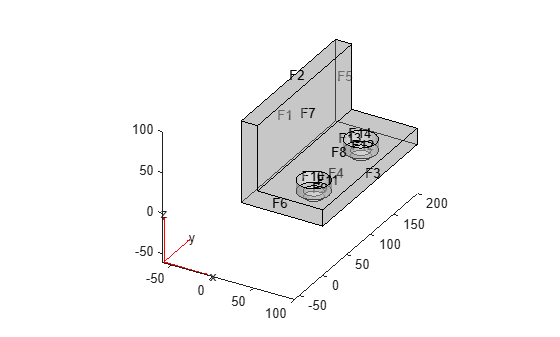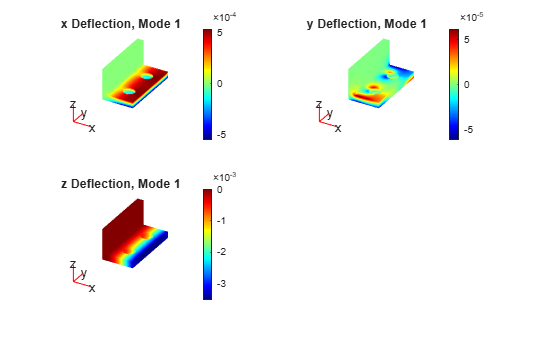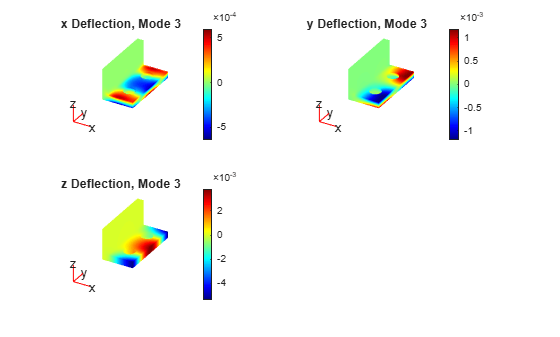# solvepdeeig

Solve PDE eigenvalue problem specified in a PDEModel

## Syntax

``result = solvepdeeig(model,evr)``

## Description

example

````result = solvepdeeig(model,evr)` solves the PDE eigenvalue problem in `model` for eigenvalues in the range `evr`. If the range does not contain any eigenvalues, `solvepdeeig` returns an `EigenResults` object with the empty `EigenVectors`, `EigenValues`, and `Mesh` properties.```

## Examples

collapse all

Solve for several vibrational modes of the `BracketTwoHoles` geometry.

The equations of elasticity have three components. Therefore, create a PDE model that has three components. Import and view the `BracketTwoHoles` geometry.

```model = createpde(3); importGeometry(model,"BracketTwoHoles.stl"); pdegplot(model,"FaceLabels","on","FaceAlpha",0.4)```Set F1, the rear face, to have zero deflection.

`applyBoundaryCondition(model,"dirichlet","Face",1,"u",[0;0;0]);`

Set the model coefficients to represent a steel bracket. For details, see Linear Elasticity Equations. When specifying the `f`-coefficient, assume that all body forces are zero.

```E = 200e9; % elastic modulus of steel in Pascals nu = 0.3; % Poisson's ratio specifyCoefficients(model,"m",0,... "d",1,... "c",elasticityC3D(E,nu),... "a",0,... "f",[0;0;0]);```

Find the eigenvalues up to `1e7`.

`evr = [-Inf,1e7];`

Mesh the model and solve the eigenvalue problem.

```generateMesh(model); results = solvepdeeig(model,evr);```

How many results did `solvepdeeig` return?

`length(results.Eigenvalues)`
```ans = 3 ```

Plot the solution on the geometry boundary for the lowest eigenvalue.

```V = results.Eigenvectors; subplot(2,2,1) pdeplot3D(model,"ColorMapData",V(:,1,1)) title("x Deflection, Mode 1") subplot(2,2,2) pdeplot3D(model,"ColorMapData",V(:,2,1)) title("y Deflection, Mode 1") subplot(2,2,3) pdeplot3D(model,"ColorMapData",V(:,3,1)) title("z Deflection, Mode 1")```Plot the solution for the highest eigenvalue.

```figure subplot(2,2,1) pdeplot3D(model,"ColorMapData",V(:,1,3)) title("x Deflection, Mode 3") subplot(2,2,2) pdeplot3D(model,"ColorMapData",V(:,2,3)) title("y Deflection, Mode 3") subplot(2,2,3) pdeplot3D(model,"ColorMapData",V(:,3,3)) title("z Deflection, Mode 3")```## Input Arguments

collapse all

PDE model, specified as a `PDEModel` object. The model contains the geometry, mesh, and problem coefficients.

Example: `model = createpde(1)`

Eigenvalue range, specified as a two-element real vector. `evr(1)` specifies the lower limit of the range of the real part of the eigenvalues, and may be `-Inf`. `evr(2)` specifies the upper limit of the range, and must be finite.

Example: `[-Inf;100]`

Data Types: `double`

## Output Arguments

collapse all

Eigenvalue results, returned as an `EigenResults` object. If the range `env` does not contain any eigenvalues, the returned `EigenResults` object has the empty `EigenVectors`, `EigenValues`, and `Mesh` properties.

## Tips

• The equation coefficients cannot depend on the solution `u` or its gradient.

## Version History

Introduced in R2016a